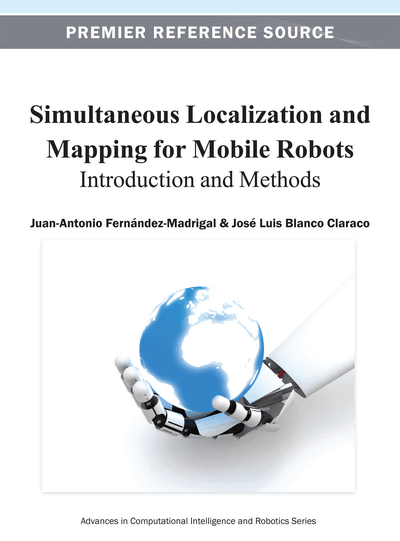# Sensor Models

Copyright: © 2013 |Pages: 29
DOI: 10.4018/978-1-4666-2104-6.ch006
OnDemand PDF Download:
Available
\$37.50
No Current Special Offers

## Abstract

This is the second chapter of the second section. Analogously to chapter 5, here the authors study probabilistic models of sensors, which is the second fundamental component of the general Bayesian framework for localization. In this chapter, they explain common mathematical models of sensors, stressing their differences and effects in further estimation techniques, in particular whether they are parametrical or not. The chapter also points out the existence of the association problem between observations and known elements of maps for some kinds of sensors, and presents solutions to that problem. Finally, some methods for matching local maps provided by particular kinds of sensors are also included.
Chapter Preview
Top

## Chapter Guideline

• You will learn:

• o

The general role of sensors in the Bayesian estimation framework for localization and mapping.

• o

The basic probabilistic models for the most important kinds of robotic sensors.

• o

The data association problem and its best known solutions.

• o

A glimpse on the different types of maps that a mobile robot can use.

• Provided tools:

• o

The NN and JCBB methods for solving the data association problem.

• o

Basic methods for matching pairs of maps.

• Relation to other chapters:

• o

The proposed probabilistic models are one piece within the proposed solutions to localization and mapping presented in chapters 7, 9, and 10.

• o

Chapter 8 provides a comprehensive overview of the different kinds of maps for mobile robotics.

Top

## 1. Introduction

In addition to modeling the uncertainty of robot actions, all Bayesian estimators employed for localization or SLAM require some mathematical model for the uncertainty introduced by sensors. In particular, before addressing mobile robot localization in chapter 7 and mapping and SLAM in chapters 8-10, we will need to provide explicit forms for, the likelihood of gathering a given observationat the end of time stepif the robot moves within a static, known or hypothetical environment, assuming an also known or hypothetical robot pose. Since current sensors do not provide topological information directly, we will assume in the following that both the robot pose and measurements are continuous (metrical) random variables.

## Complete Chapter List

Search this Book:
Reset